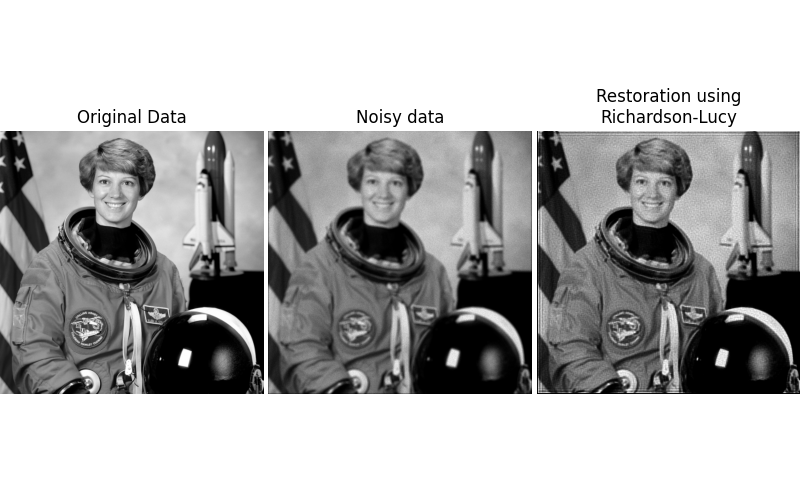# Image Deconvolution#

In this example, we deconvolve an image using Richardson-Lucy deconvolution algorithm (, ).

The algorithm is based on a PSF (Point Spread Function), where PSF is described as the impulse response of the optical system. The blurred image is sharpened through a number of iterations, which needs to be hand-tuned.```import numpy as np
import matplotlib.pyplot as plt

from scipy.signal import convolve2d as conv2

from skimage import color, data, restoration

rng = np.random.default_rng()

astro = color.rgb2gray(data.astronaut())

psf = np.ones((5, 5)) / 25
astro = conv2(astro, psf, 'same')
astro_noisy = astro.copy()
astro_noisy += (rng.poisson(lam=25, size=astro.shape) - 10) / 255.

# Restore Image using Richardson-Lucy algorithm
deconvolved_RL = restoration.richardson_lucy(astro_noisy, psf, num_iter=30)

fig, ax = plt.subplots(nrows=1, ncols=3, figsize=(8, 5))
plt.gray()

for a in (ax, ax, ax):
a.axis('off')

ax.imshow(astro)
ax.set_title('Original Data')

ax.imshow(astro_noisy)
ax.set_title('Noisy data')

ax.imshow(deconvolved_RL, vmin=astro_noisy.min(), vmax=astro_noisy.max())
ax.set_title('Restoration using\nRichardson-Lucy')